# Anatomy of gghighlight

gghighlight
ggplot2
Author

Hiroaki Yutani

Published

June 3, 2018

I’m overhauling my gghighlight package toward the upcoming release of ggplot2 2.3.0. I think I will introduce about the new `gghighlight()` soon, but before that, I want to write out the ideas behind gghighlight.

Note that what I’ll write here contains few new things, as the basic idea is already covered by this great post:

My post is mainly for organizing my thought, yet I hope someone find this useful :)

``````# tweak for plotting
knit_print.ggplot <- function(x, ...) {
x <- x  +
theme_minimal() +
scale_x_continuous(expand = expand_scale(mult = 0.5)) +
scale_y_continuous(expand = expand_scale(mult = 0.2))
ggplot2:::print.ggplot(x, ...)
}``````

## Data

Suppose we have this data:

x y type value
3 3 a 0
8 3 a 1
13 3 a 0
2 2 b 0
7 2 b 10
12 2 b 10
1 1 c 10
6 1 c 20
11 1 c 0

## Simple plot

If we plot the data very simply, the code would be like this:

``````library(tidyverse)

ggplot(d, aes(x, y, colour = type)) +
geom_point(size = 10)``````
``````Warning: `expand_scale()` was deprecated in ggplot2 3.3.0.
This warning is displayed once every 8 hours.
Call `lifecycle::last_lifecycle_warnings()` to see where this warning was generated.``````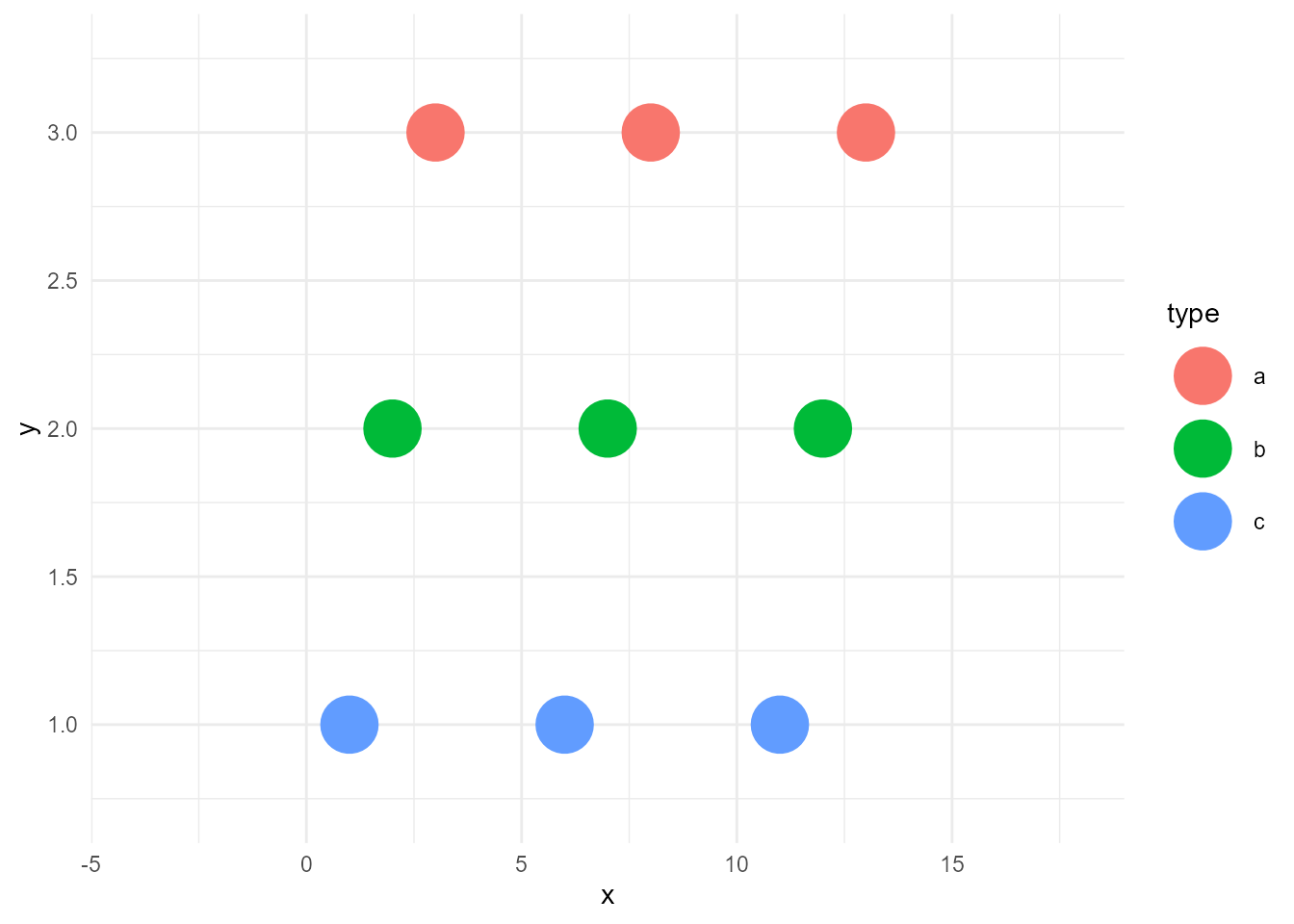## Highlighted plot

Now, what if we want to highlight only the points of records whose `type` are `"b"`?

We need two layers:

1. unhighlighted layer
2. highlighted layer

### Create an unhighlighted layer

An unhighlighted layer is the colorless version of the above points with the same data. To create this, we can simply remove `colour` from `aes()` and specify a static colour `"grey"`. I call this operation as bleach.

``````bleached_layer <- geom_point(data = d, aes(x, y),
size = 10, colour = "grey")``````

If we plot this, the result would be below:

``````ggplot() +
bleached_layer``````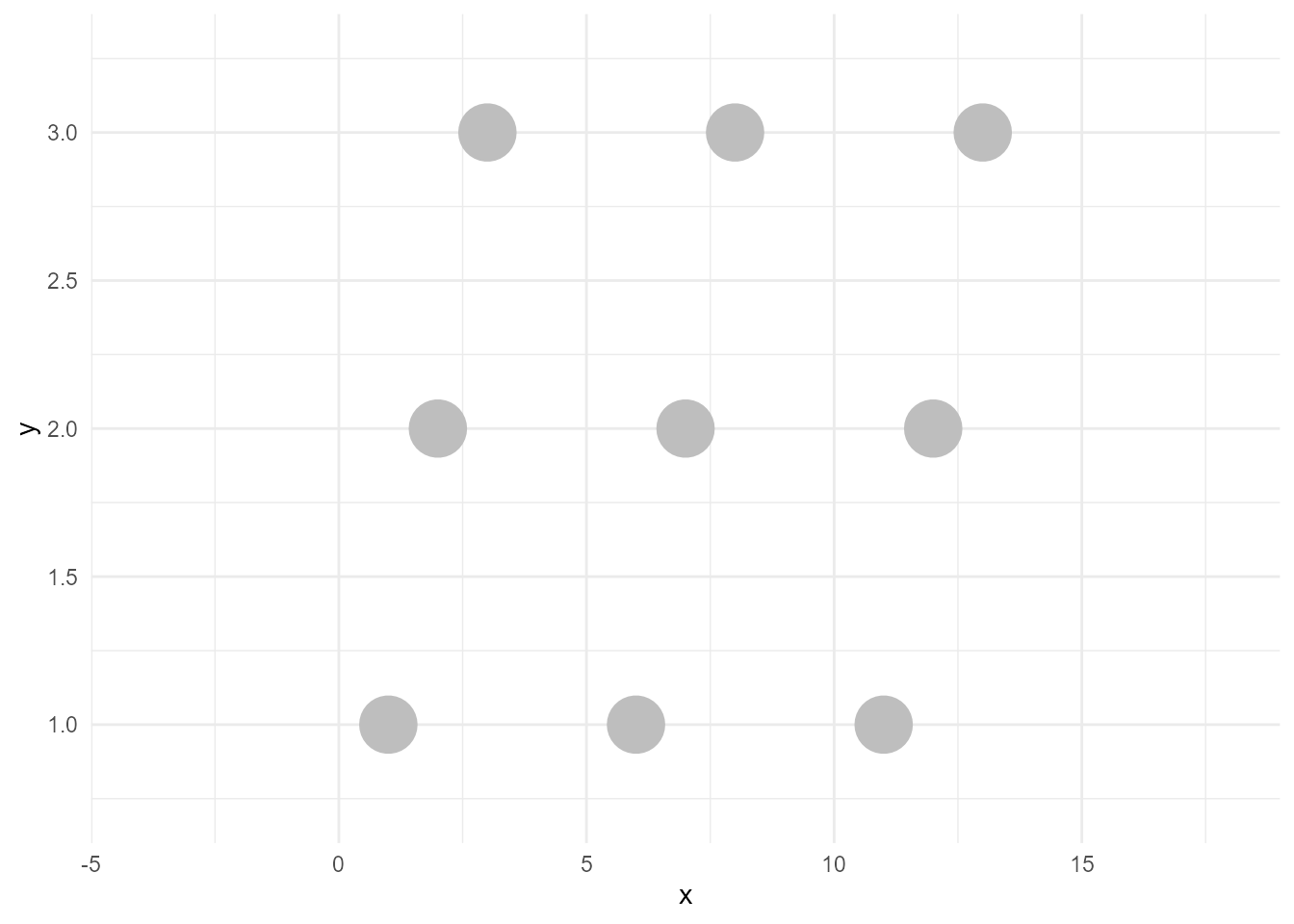### Create a highlighted layer

A highlighted layer is the fewer-data version of the above points with (not necessarily the same) colors. To create this, we need some data manipulation. Let’s filter the data.

``d_sieved <- filter(d, type == "b")``

Then the layer we want can be created like below. I call this operation as sieve (filter might be a better word, but I wanted to choose another word than dplyr’s verbs to avoid confusion).

``````sieved_layer <- geom_point(data = d_sieved, aes(x, y, colour = type),
size = 10)``````

If we plot this, the result would be below:

``````ggplot() +
sieved_layer``````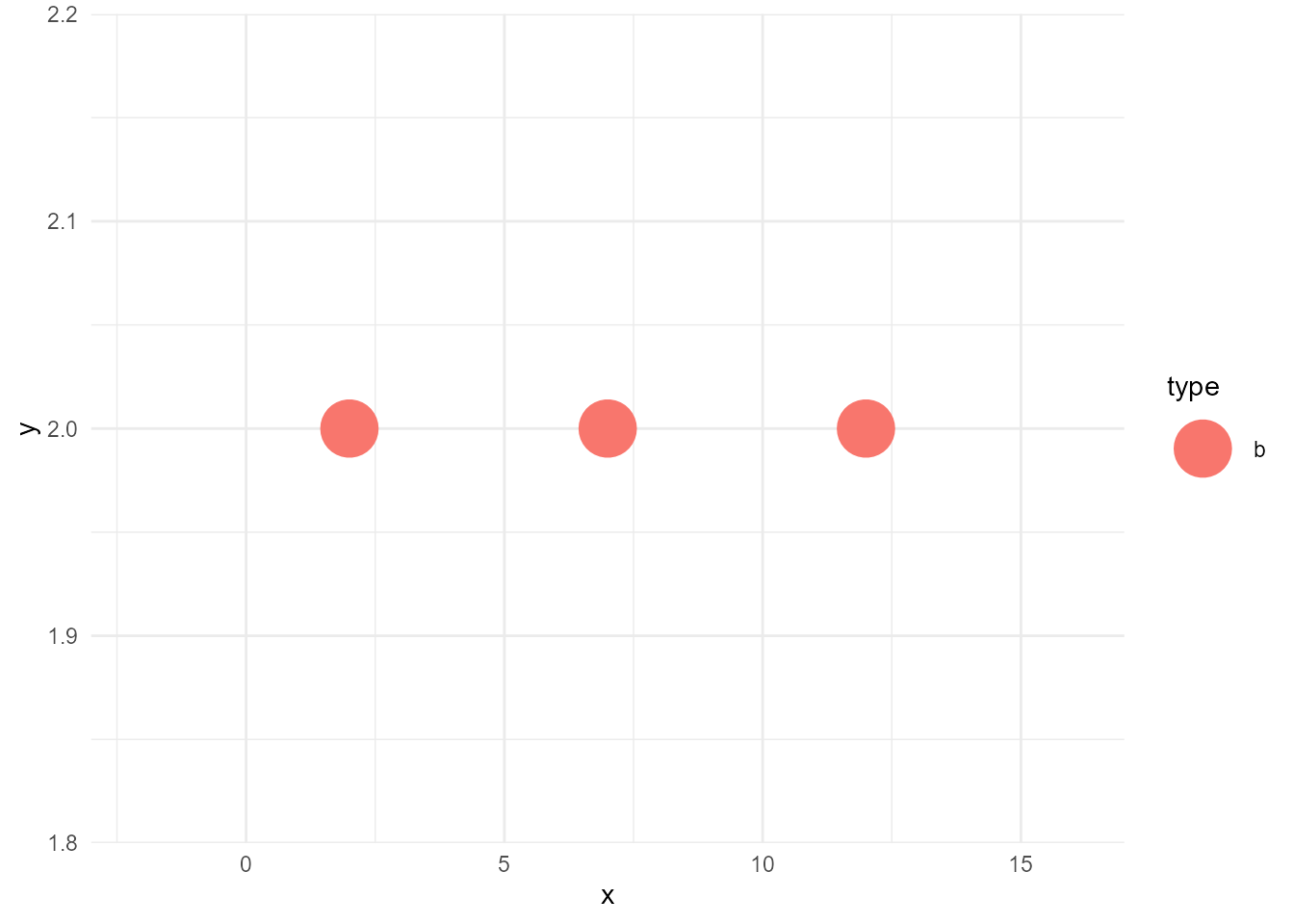### Join the two layers

Now we can draw the highlighted version of the plot as below:

``````ggplot() +
bleached_layer +
sieved_layer``````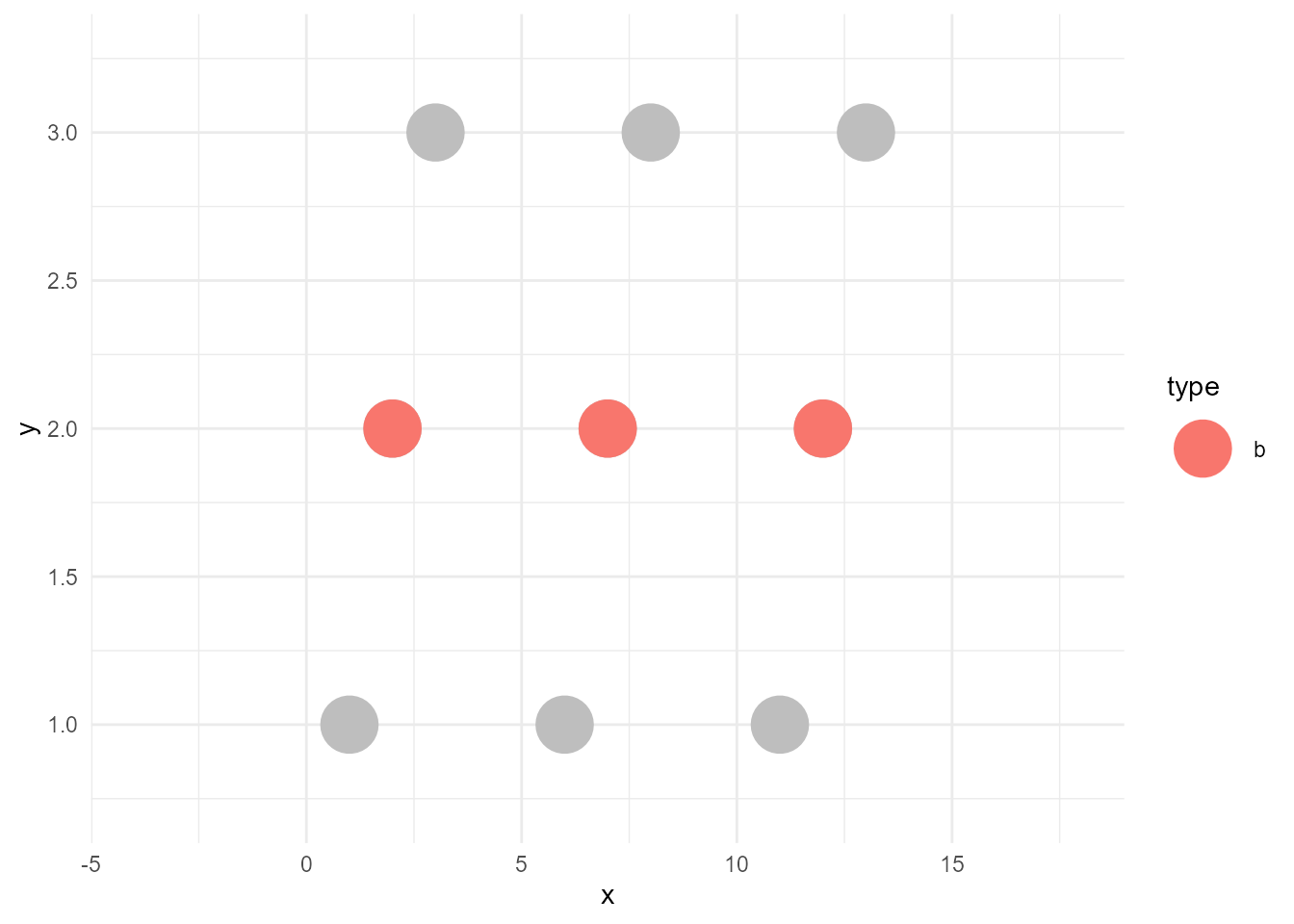## “by point” vs “by group”

So far, so good. Then, let’s consider a bit about the case when the geom is not point, but line.

While points can be plotted one by one, lines cannot be drawn without the relationship between points. For example, haven’t you experienced an unexpected zigzag line?

``````ggplot(d, aes(x, y)) +
geom_line(size = 3)``````
``````size aesthetic has been deprecated for use with lines as of ggplot2 3.4.0
This message is displayed once every 8 hours.``````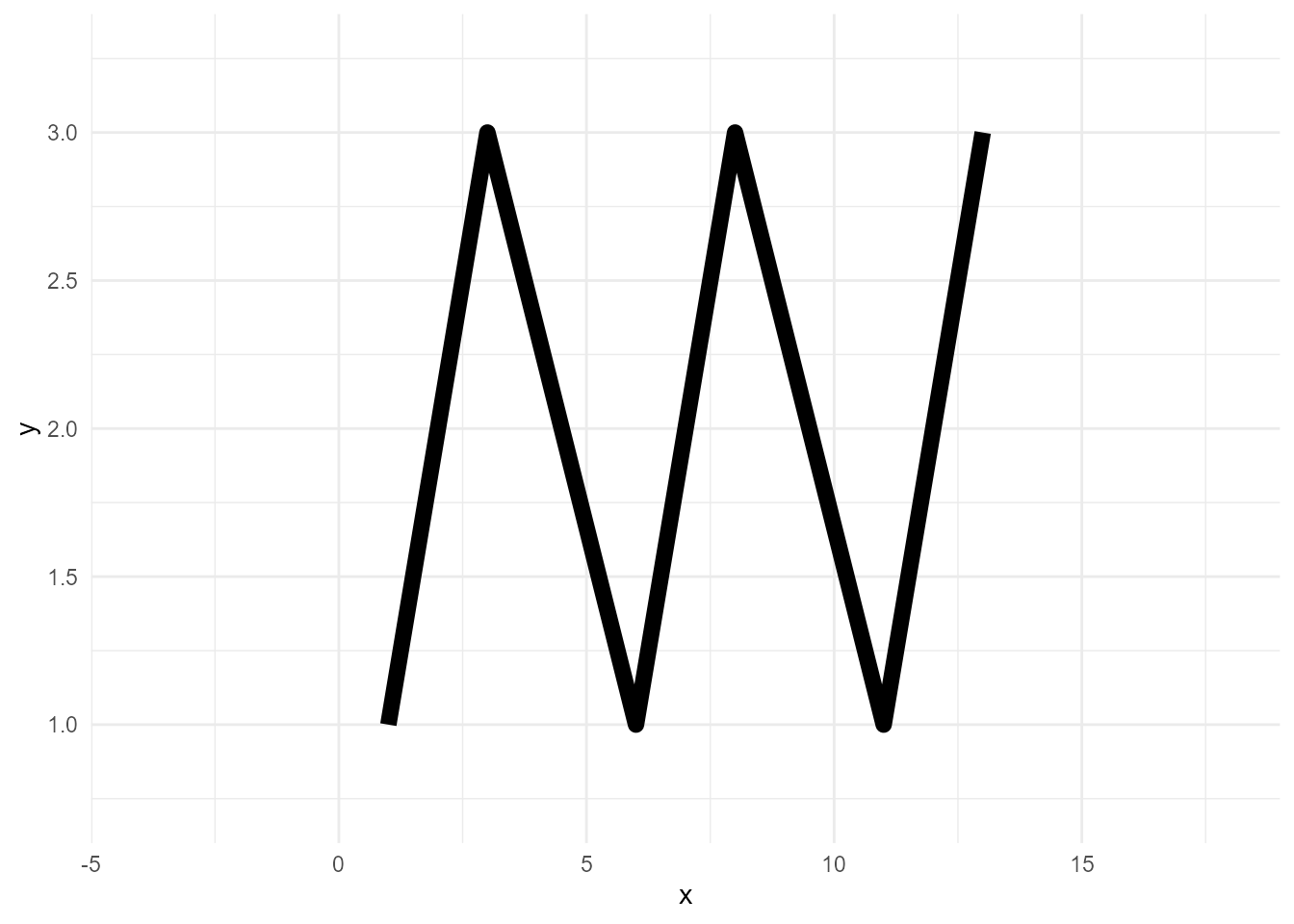Lines need `group` variable, which indicates the series of data points.

``````ggplot(d, aes(x, y, group = type)) +
geom_line(size = 3)``````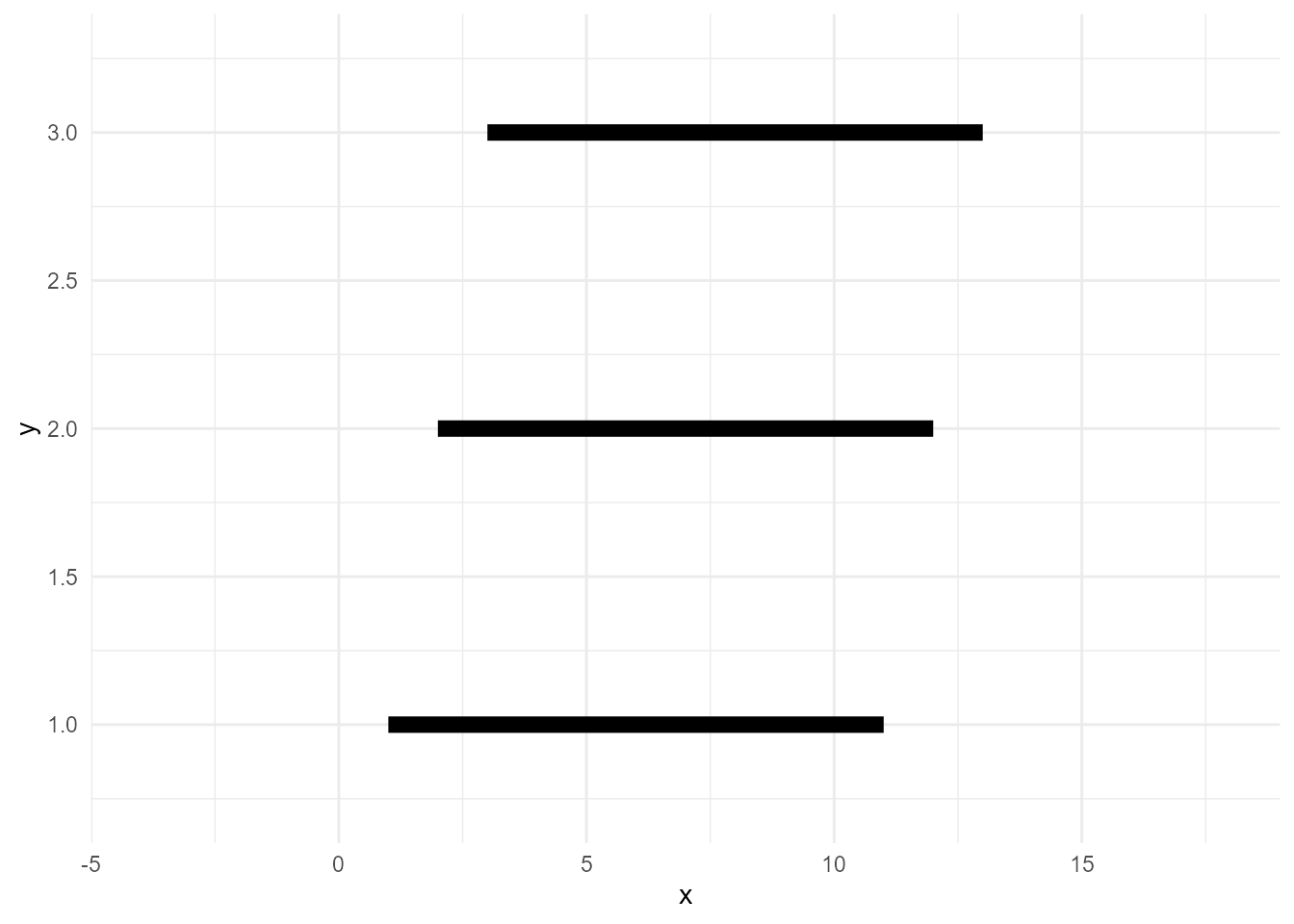Note that `group` doesn’t need to be declared explicitly, as ggplot2 infers the groups from the specified variables. More precisely, it calculates group IDs based on the combination of discrete variables here. So, usually, specifying a discrete variable on `colour` or `fill` is enough.

``````ggplot(d, aes(x, y, colour = type)) +
geom_line(size = 3)``````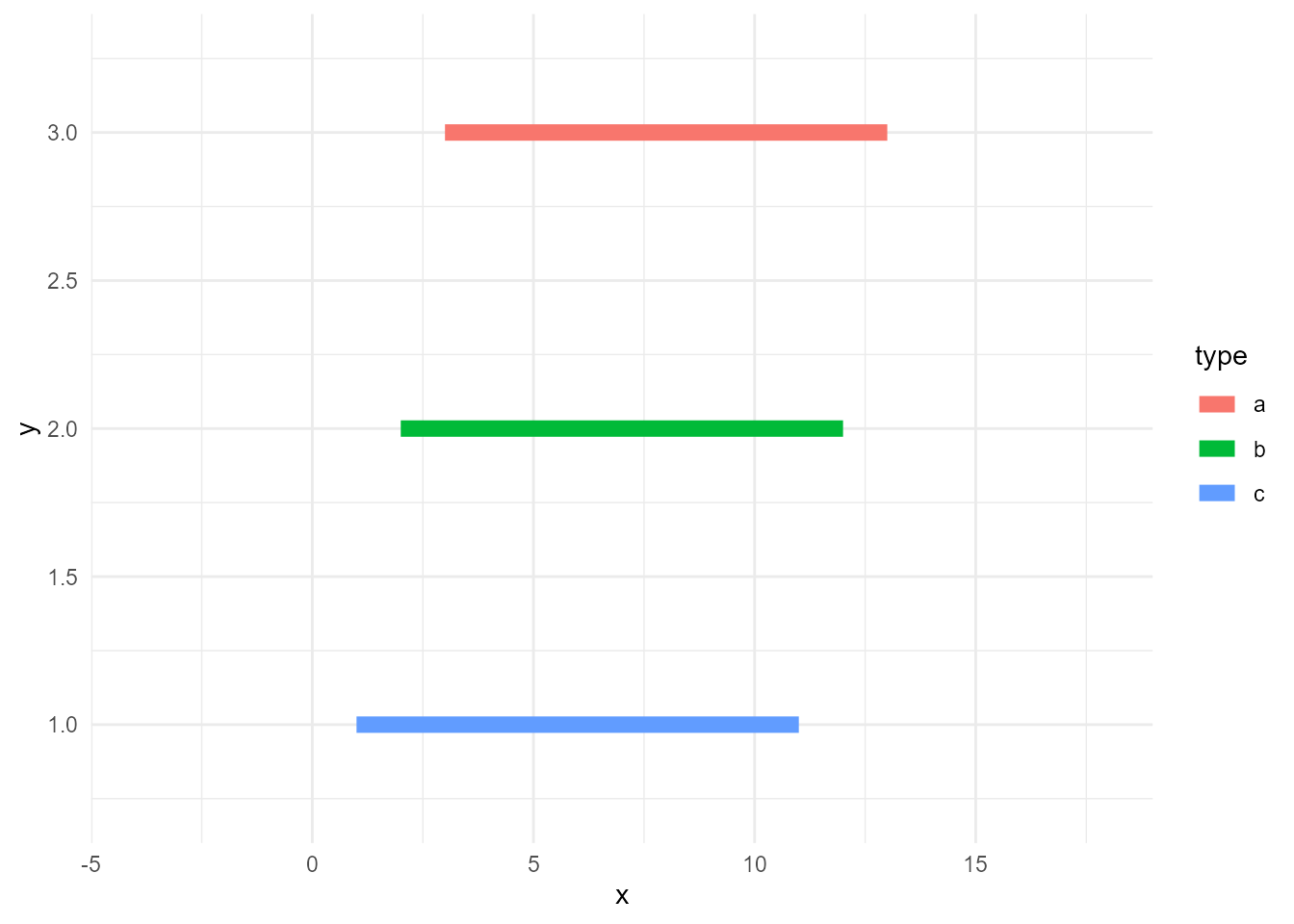Anyway, lines need groups. Accordingly, we need to consider the group when we sieve the data. Otherwise, the lines will be incomplete as this example:

``````# data whose values are >=10
d_sieved2 <- filter(d, value >= 10)

ggplot() +
geom_line(data = d, aes(x, y, group = type), size = 3, colour = "grey") +
geom_line(data = d_sieved2, aes(x, y, colour = type), size = 3)``````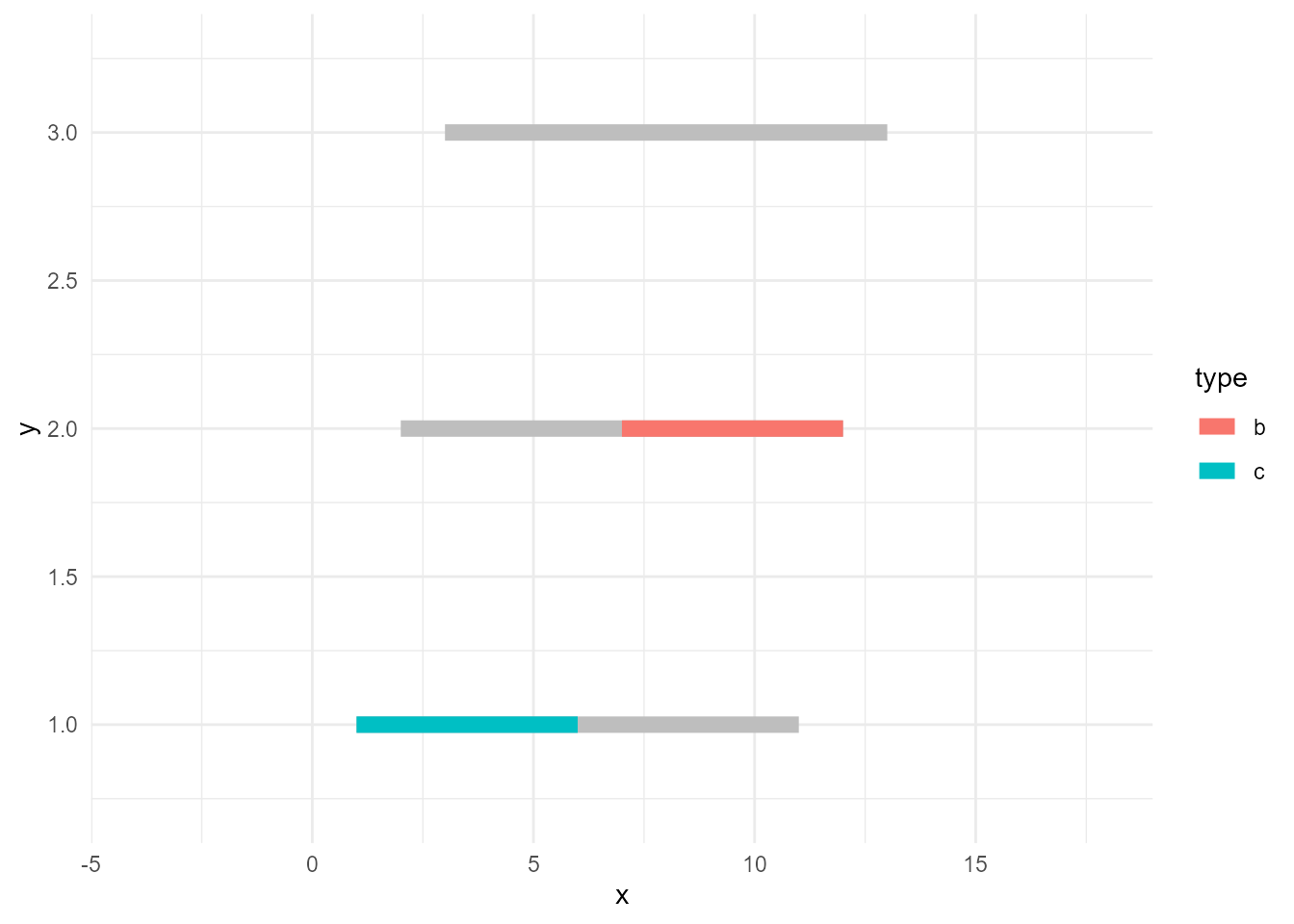So, the correct way of doing this is to use `group_by()` and some aggregate functions like `max()` so that the calculations are done by group.

``````# data series whose max values are >=10
d_sieved3 <- d %>%
group_by(type) %>%
filter(max(value) >= 10)

ggplot() +
geom_line(data = d, aes(x, y, group = type), size = 3, colour = "grey") +
geom_line(data = d_sieved3, aes(x, y, colour = type), size = 3)``````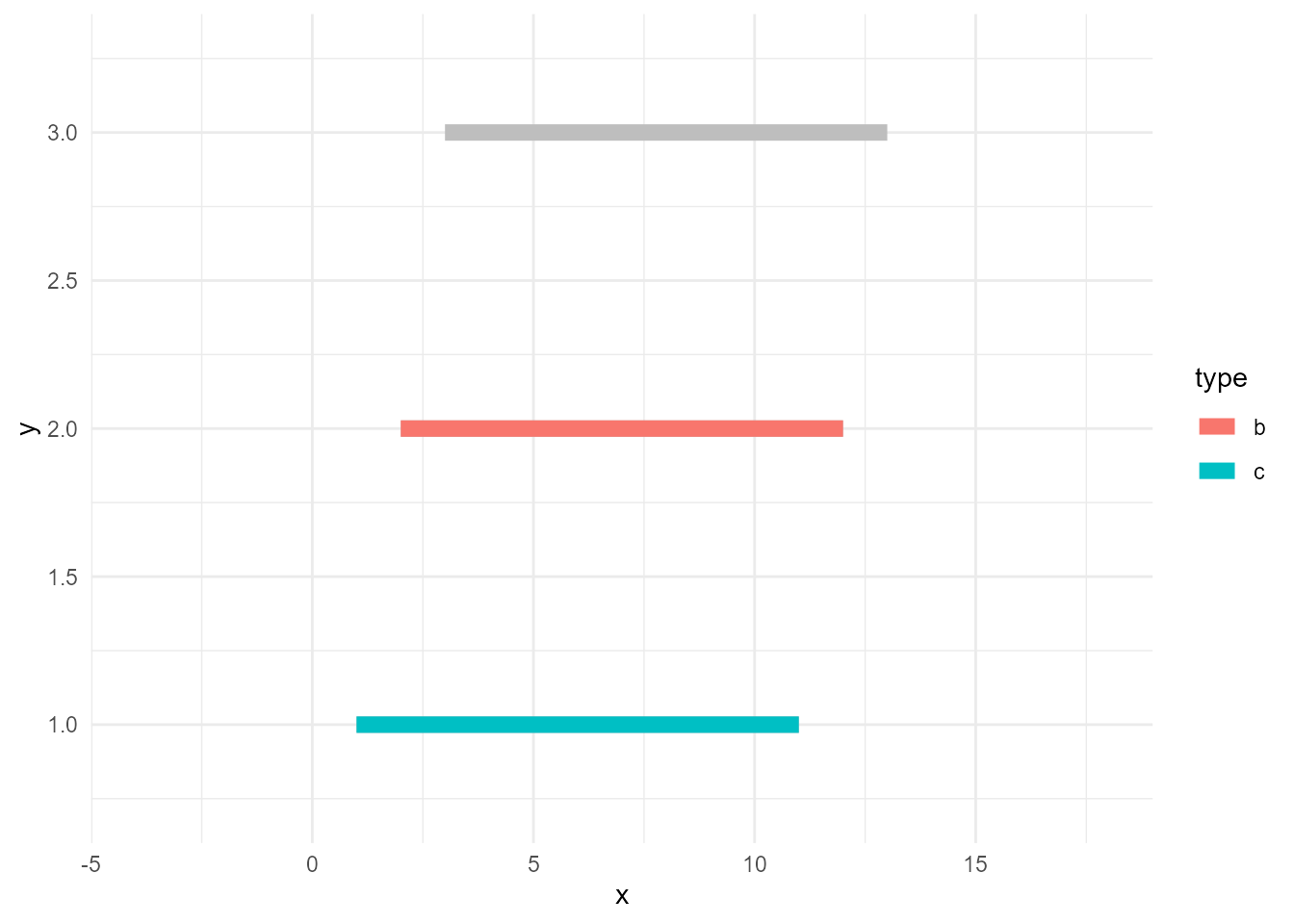## Prevent unhighlighted layer from facetted

Next topic is facetting. Let’s naively facet the plot above.

``````ggplot() +
geom_line(data = d, aes(x, y, group = type), size = 3, colour = "grey") +
geom_line(data = d_sieved3, aes(x, y, colour = type), size = 3) +
facet_wrap(~ type)``````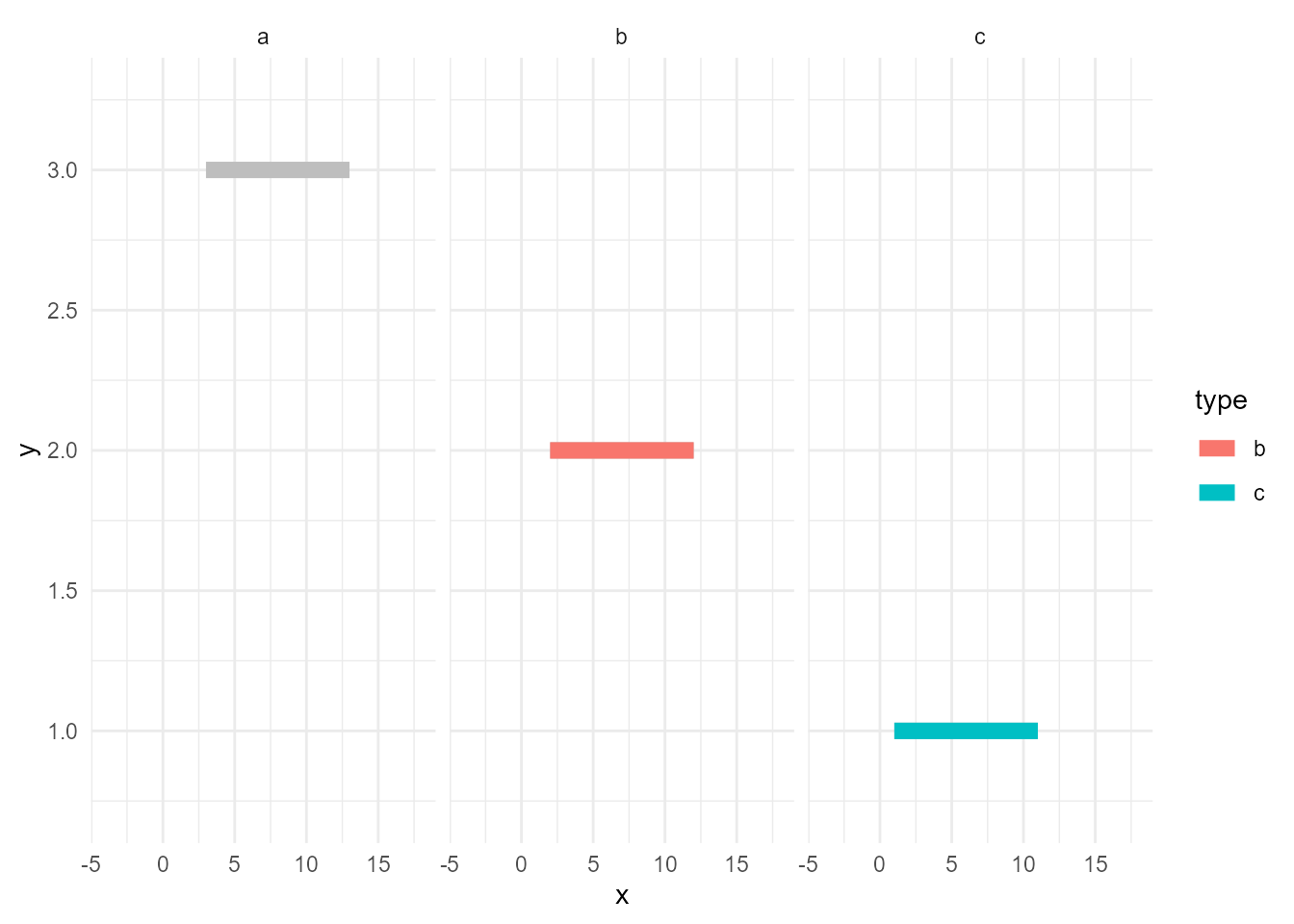Hmm…, unhighlighted lines are facetted. But, maybe we want the grey ones exists in all of the panels.

`facet_*()` facets all layers if the data contains the specified variable, in this case `type`. In other words, if the layer’s data doesn’t have the variable, it won’t get facetted. Let’s rename it.

``````d_bleached <- d
names(d_bleached) <- "GROUP"

ggplot() +
geom_line(data = d_bleached, aes(x, y, group = GROUP), size = 3, colour = "grey") +
geom_line(data = d_sieved3, aes(x, y, colour = type), size = 3) +
facet_wrap(~ type)``````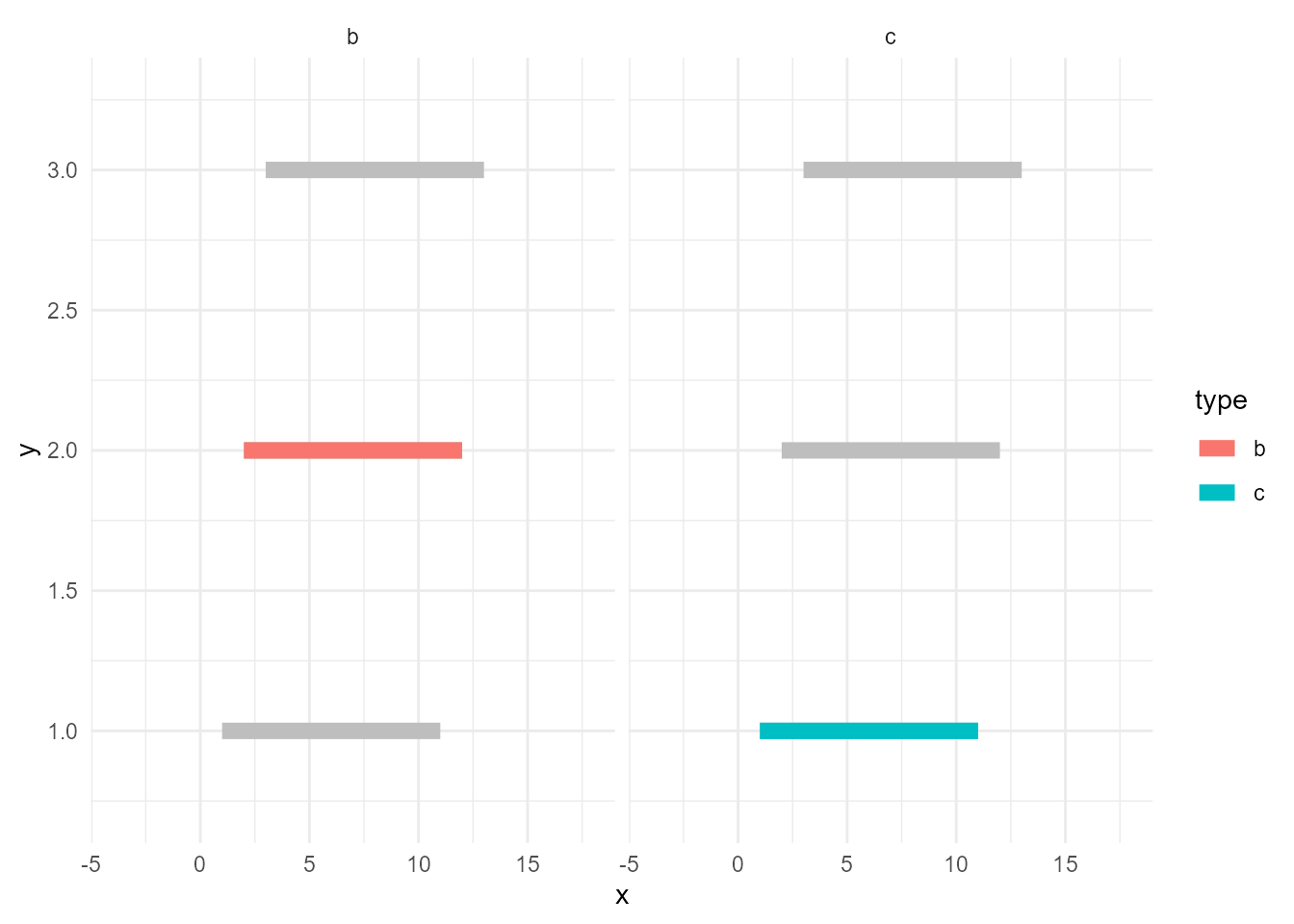You may notice about one more good thing; the panel for `"a"` disappeared. This is because now `d_sieved3` is the only data that contains `type` and it has only records of `"b"` and `"c"`.

## Some spoilers

The next version of gghighlight will do the above things almost automatically. All you have to do is just adding `gghighlight()`.

``````library(gghighlight)

ggplot(d, aes(x, y, colour = type)) +
geom_point(size = 10) +
gghighlight(type == "b")``````
``````Warning: Tried to calculate with group_by(), but the calculation failed.
Falling back to ungrouped filter operation...``````
``label_key: type``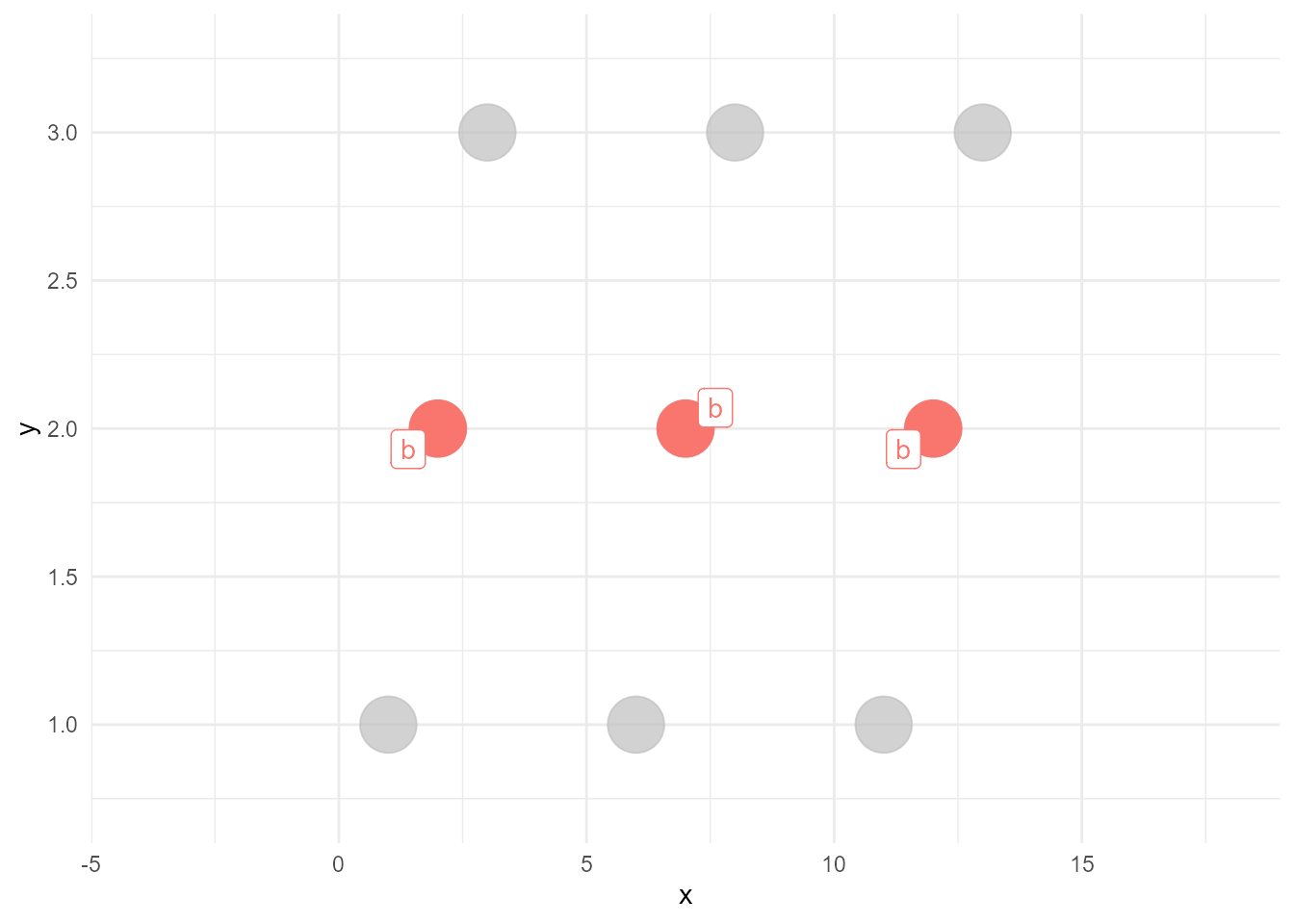``````ggplot(d, aes(x, y, colour = type)) +
geom_line(size = 3) +
gghighlight(max(value) >= 10)``````
``label_key: type``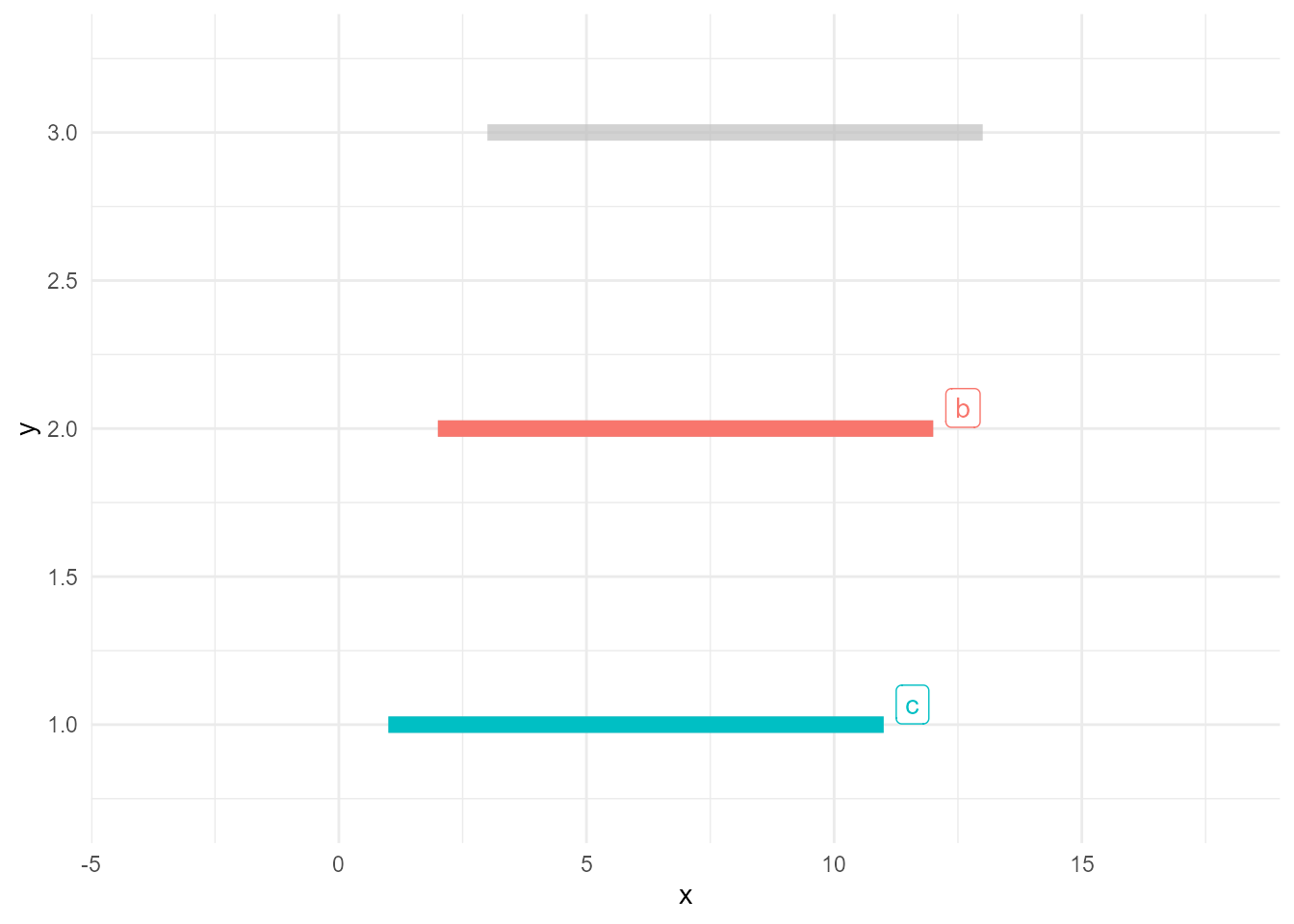Stay tuned!